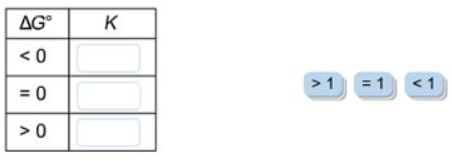# Problem: Complete this table relating the values of ΔG° and K.

###### FREE Expert Solution

Recall that ΔG˚rxn and K are related to each other:

$\overline{){\mathbf{\Delta G}}{{\mathbf{°}}}_{{\mathbf{rxn}}}{\mathbf{=}}{\mathbf{-}}{\mathbf{RTlnK}}}$

Recall that if:

• ΔG < 0 or ΔG = (–); the reaction is spontaneous

• ΔG = 0; the reaction is at equilibrium

• ΔG > 0 or ΔG = (+); the reaction is non-spontaneous

When:

K = 1

85% (144 ratings)###### Problem Details

Complete this table relating the values of ΔG° and K.